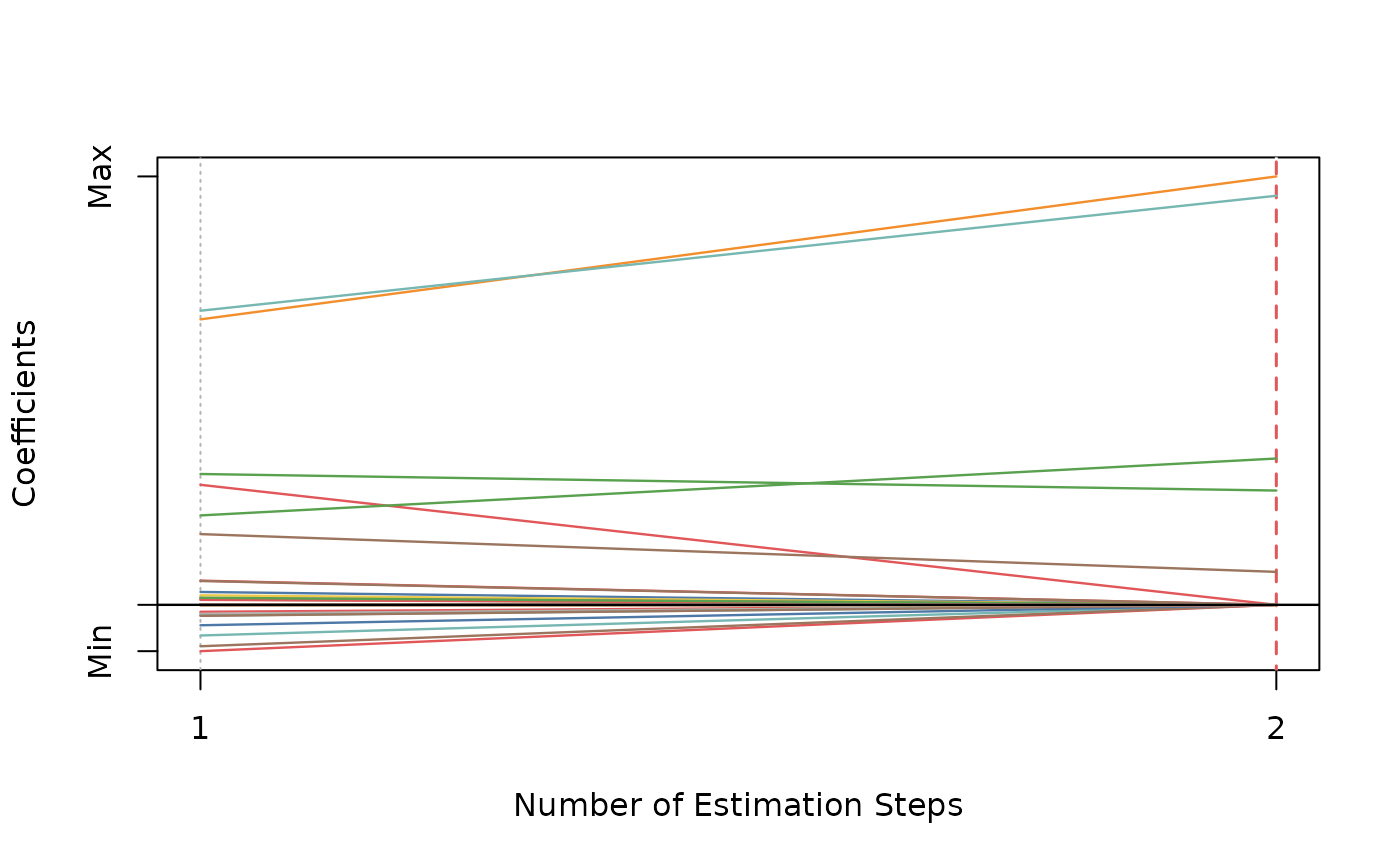## Usage

amnet(
x,
y,
family = c("gaussian", "binomial", "poisson", "cox"),
init = c("mnet", "ridge"),
gammas = 3,
alphas = seq(0.05, 0.95, 0.05),
tune = c("cv", "ebic", "bic", "aic"),
nfolds = 5L,
ebic.gamma = 1,
scale = 1,
eps = 1e-04,
max.iter = 10000L,
penalty.factor.init = rep(1, ncol(x)),
seed = 1001,
parallel = FALSE,
verbose = FALSE
)

## Arguments

x

Data matrix.

y

Response vector if family is "gaussian", "binomial", or "poisson". If family is "cox", a response matrix created by Surv.

family

Model family, can be "gaussian", "binomial", "poisson", or "cox".

init

Type of the penalty used in the initial estimation step. Can be "mnet" or "ridge".

gammas

Vector of candidate gammas (the concavity parameter) to use in MCP-Net. Default is 3.

alphas

Vector of candidate alphas to use in MCP-Net.

tune

Parameter tuning method for each estimation step. Possible options are "cv", "ebic", "bic", and "aic". Default is "cv".

nfolds

Fold numbers of cross-validation when tune = "cv".

ebic.gamma

Parameter for Extended BIC penalizing size of the model space when tune = "ebic", default is 1. For details, see Chen and Chen (2008).

scale

Scaling factor for adaptive weights: weights = coefficients^(-scale).

eps

Convergence threshhold to use in MCP-net.

max.iter

Maximum number of iterations to use in MCP-net.

penalty.factor.init

The multiplicative factor for the penalty applied to each coefficient in the initial estimation step. This is useful for incorporating prior information about variable weights, for example, emphasizing specific clinical variables. To make certain variables more likely to be selected, assign a smaller value. Default is rep(1, ncol(x)).

seed

Random seed for cross-validation fold division.

parallel

Logical. Enable parallel parameter tuning or not, default is FALSE. To enable parallel tuning, load the doParallel package and run registerDoParallel() with the number of CPU cores before calling this function.

verbose

Should we print out the estimation progress?

## Value

List of model coefficients, ncvreg model object, and the optimal parameter set.

## Author

Nan Xiao <https://nanx.me>

## Examples

dat <- msaenet.sim.gaussian(
n = 150, p = 500, rho = 0.6,
coef = rep(1, 5), snr = 2, p.train = 0.7,
seed = 1001
)

amnet.fit <- amnet(
dat$x.tr, dat$y.tr,
alphas = seq(0.2, 0.8, 0.2), seed = 1002
)
#> Warning: ncvreg() is intended for pathwise optimization, not for single values of lambda.
#>   1. You are strongly encouraged to fit a path and extract the solution at the lambda value of interest, rather than use ncvreg() in this way.
#>   2. In particular, if you are using the MCP or SCAD penalties, be aware that you greatly increase your risk of converging to an inferior local maximum if you do not fit an entire path.
#>   3. You may wish to look at the ncvfit() function, which is intended for non-path (i.e., single-lambda) optimization and allows the user to supply initial values.
#> Warning: ncvreg() is intended for pathwise optimization, not for single values of lambda.
#>   1. You are strongly encouraged to fit a path and extract the solution at the lambda value of interest, rather than use ncvreg() in this way.
#>   2. In particular, if you are using the MCP or SCAD penalties, be aware that you greatly increase your risk of converging to an inferior local maximum if you do not fit an entire path.
#>   3. You may wish to look at the ncvfit() function, which is intended for non-path (i.e., single-lambda) optimization and allows the user to supply initial values.

print(amnet.fit)
#> Call: amnet(x = dat$x.tr, y = dat$y.tr, alphas = seq(0.2, 0.8, 0.2),
#>     seed = 1002)
#>   Df    Lambda Gamma Alpha
#> 1  5 0.2826426     3   0.8
msaenet.nzv(amnet.fit)
#>    2   4   5  35 269
msaenet.fp(amnet.fit, 1:5)
#>  2
msaenet.tp(amnet.fit, 1:5)
#>  3
amnet.pred <- predict(amnet.fit, dat$x.te) msaenet.rmse(dat$y.te, amnet.pred)
#>  2.67195
plot(amnet.fit)